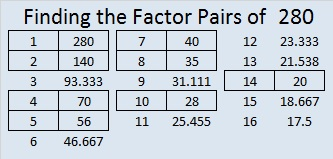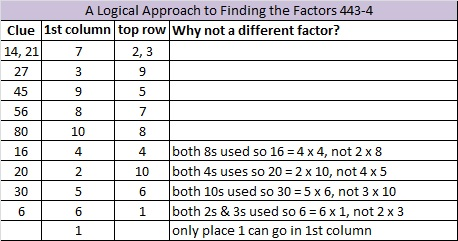# 280 and Level 4

• 280 is a composite number.
• Prime factorization: 280 = 2 x 2 x 2 x 5 x 7, which can be written 280 = 2³ x 5 x 7
• The exponents in the prime factorization are 3, 1, and 1. Adding one to each and multiplying we get (3 + 1)(1 + 1)(1 + 1) = 4 x 2 x 2 = 16. Therefore 280 has 16 factors.
• Factors of 280: 1, 2, 4, 5, 7, 8, 10, 14, 20, 28, 35, 40, 56, 70, 140, 280
• Factor pairs: 280 = 1 x 280, 2 x 140, 4 x 70, 5 x 56, 7 x 40, 8 x 35, 10 x 28, or 14 x 20
• Taking the factor pair with the largest square number factor, we get √280 = (√4)(√70) = 2√70 ≈ 16.733When I was a child, my brother showed me how he could multiply using his fingers. The same method is clearly illustrated here. If you haven’t seen it before, take a look. It isn’t the fastest method for multiplying, but it is still fun to see that it works. You can also practice the multiplication facts by finding the factors that solve this puzzle and then completing the multiplication table.Print the puzzles or type the factors on this excel file: 10 Factors 2014-10-27This site uses Akismet to reduce spam. Learn how your comment data is processed.